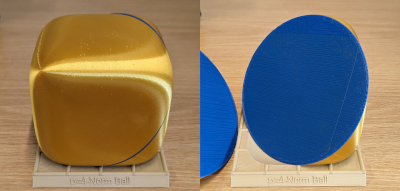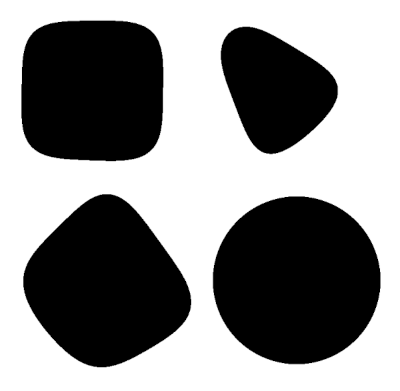# Random slices of a sphube

Ben Grimmer posted something yesterday on Twitter:

A nice mathematical puzzle🧐

If you take a 4-norm ball and cut it carefully, you will find a two-norm ball. 3D printed visual evidence below.
The puzzle: Why does this happen and how much more generally does it happen?

(This question was first posed to me by Pablo Parrilo)This is a surprising result, and it touches on two things I’ve written about before.

Several years ago I wrote about the surprising result that if you slice open a Menger sponge a certain way then you can see six-pointed stars. I was just as surprised to see the result above. I also wrote several posts about “squircles,” and the 4-norm ball is a 3D version of the squircle, sometimes called a “sphube.”

I modified the code I’d written for the Menger sponge post to create random slides of the 4-norm ball. Here are some of the results.One of these happens to be a circle, or at least close enough that it looks like a circle.

My code generated a random point and a random normal vector to determine a plane through that point. That’s how I produced the square-ish and triangle-ish plots.

For the last image, I set the normal vector to (1, 1, 1) because the 3D printed image above suggested that would be the right direction. I generated random points inside the cube, and one time the random point was approximately (0.2, 0, -0.2) and noticed the plot was approximately circular. It seems that when z = −x and the normal vector is (1, 1, 1) you get a circular cross section. By symmetry you can make many more examples from this example.

I have not proved anything yet; just played with plots.

## 2 thoughts on “Random slices of a sphube”

1. Francois Glineur

If we cut with the z=x+y plane, we find that x^4+y^4+z^4 is equal to
x^4+y^4+(x+y)^4 = 2(x²+xy+y²)² = (x²+y²+z²)²/2
Hence this slice of the unit 4-norm ball is a disk with radius √2.

More generally, one can identify four suitable cuts using identity:
2(x^4 +y^4+z^4) – (x²+y²+z²)² = (x-y-z)(x+y-z)(x-y+z)(x+y+z)

2. Oliver Jennrich

A quick check w/ Mathematica shows that your suspicion is true – if you cut the sphube by a plane w/ normal vector n=(1,1,1) and a point on the line z+x=0, the resulting figure is a circle. Note that in this case the equation of the plane is r.n=0. (as (-a,0,a).(1,1,1)=0). So the plane goes through the origin.

Rotating the coordinate system such that the plane is the X-Y plane, the intersection between the sphube and the plane takes the form X^2+Y^2=√2.